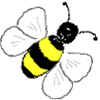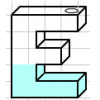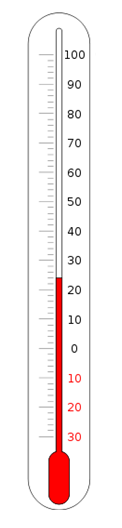#### You may also like### Speedy Sidney

Two trains set off at the same time from each end of a single straight railway line. A very fast bee starts off in front of the first train and flies continuously back and forth between the two trains. How far does Sidney fly before he is squashed between the two trains?### Air Nets

Can you visualise whether these nets fold up into 3D shapes? Watch the videos each time to see if you were correct.### Maths Filler

Imagine different shaped vessels being filled. Can you work out what the graphs of the water level should look like?

# Temperature

##### Age 11 to 14Challenge LevelTemperature printable worksheet

Temperature is often measured in degrees Celsius,$^\circ C$, or degrees Fahrenheit,$^\circ F$.

The freezing point of water is $0^\circ$C and $32^\circ$F.
The boiling point of water is $100^\circ$C and $212^\circ$F.

Is there a temperature at which Celsius and Fahrenheit readings are the same?

Can you describe a way of converting Fahrenheit readings into Celsius?

Can you describe a way of converting Celsius readings into Fahrenheit?